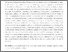# Diffraction of flexural-gravity waves by a vertical cylinder of non-circular cross section

Dişibüyük, N. B., and Yılmaz, O. (2020) Diffraction of flexural-gravity waves by a vertical cylinder of non-circular cross section. Applied Ocean Research, 101. ISSN 0141-1187Preview PDF (Accepted_Manuscript) - Accepted Version Available under License Creative Commons Attribution Non-commercial No Derivatives. Download (1MB) | Preview

## Abstract

The linear three-dimensional problem of flexural-gravity wave (hydro-elastic wave) diffraction by a vertical cylinder of an arbitrary smooth cross section is studied using an asymptotic approach combined with the vertical mode method for water of finite depth. The surface of the water is covered by an infinite, continuous elastic ice plate. The rigid cylinder extends from the sea bottom to the ice surface. The ice plate is frozen to the cylinder. The ice deflection is described by the equation of a thin elastic plate of constant thickness with clamped edge conditions at the cylinder. The flow under the ice is described by the linear theory of potential flows. The coupled problem of wave diffraction is solved in two steps. First, the problem is solved without evanescent waves similar to the problem of water waves diffracted by a vertical cylinder. This solution does not satisfy the edge conditions. Second, a radiation problem with a prescribed motion of the ice plate edge is solved by the vertical mode method. The sum of these two solutions solve the original problem. Both solutions are obtained by an asymptotic method with a small parameter quantifying a small deviation of the cylinder cross section from a circular one. Third-order asymptotic solutions are obtained by solving a set of two-dimensional boundary problems for Helmholtz equations in the exterior of a circle. Strains along the edge, where the ice plate is frozen to the cylinder, are investigated for nearly square and elliptic cross sections of the vertical cylinders depending on the characteristics of ice and incident wave. The strains are shown to be highest in the places of high curvatures of the cross sections. The derived asymptotic formulae can be used in design of vertical columns in ice. They directly relate the strains in ice plate to the shape of the column.

Item Type: Article asymptotic approach,clamped edge conditions,hydro-elastic waves,ice cover,non-circular vertical cylinder,vertical mode method,ocean engineering ,/dk/atira/pure/subjectarea/asjc/2200/2212 Faculty of Science > School of Mathematics Faculty of Science > Research Groups > Fluid and Solid Mechanics http://www.scopus.com/inward/record.url?... LivePure Connector 22 Aug 2020 00:03 22 Oct 2022 06:37 https://ueaeprints.uea.ac.uk/id/eprint/76539 10.1016/j.apor.2020.102234View Item